# CLASS 9 MATH NCERT SOLUTION FOR CHAPTER – 9 AREAS OF PARALLELOGRAMS AND TRIANGLES EX – 9.4

## Areas of Parallelograms and Triangles

Question 1.
Parallelogram ABCD and rectangle ABEF are on the same base AB and have equal areas. Show that the perimeter of the parallelogram is greater than that of the rectangle.

Solution.
We have a parallelogram ABCD and rectangle ABEF such that
ar (parallelogram ABCD) = ar (rectangle ABEF)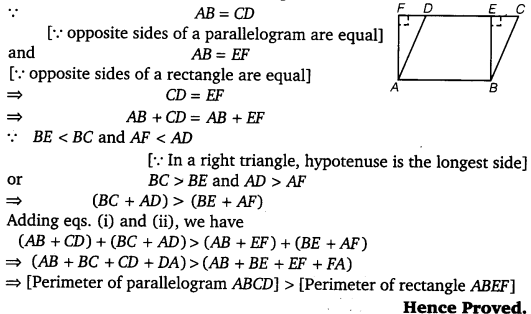Question 2.
In figure, D and E are two points on BC such that BD = DE = EC. Show that
ar (Δ ABD) = ar (Δ ADE) = ar (Δ  AEC).
Can you now answer the question that you have left in the ‘Introduction’ of this chapter, whether the field of Budhia has been actually ‘ divided into three parts of equal area?

[Remark: Note that by taking BD = DE = EC, the triangle ABC is divided into three triangles ABD, ADE and AEC of equal areas. In the same way, by dividing BC into n equal parts and joining the points of division so obtained to the opposite vertex of BC, you can divide Δ ABC into n triangles of equal areas.]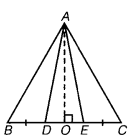Solution.
Given : ABC is a triangle, D and E are two points on BC, such that BD = DE – EC.
To prove :     ar (Δ  ABD) = ar (Δ ADE) = ar (Δ AEC)
Proof: Let AO be the perpendicular to BC.
We know that,Question 3.
In the figure, ABCD, DCFE and ABFE are parallelograms.Show that ar (Δ ADE) = ar (Δ BCF).

Solution.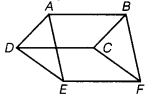Given : ABCD, DCFE and ABFE are parallelograms.
To prove : ar (Δ ADE) = ar (Δ BCE)Question 4.
In the figure, ABCD is a parallelogram and BC is produced to a point Q such that AD = CQ. If AQ intersects DC at P, then show that ar (Δ BPO = ar (Δ DPQ.) [Hint: Join AC]

Solution.Question 5.
In the figure, ABC and BDE are two equilateral triangles such that D is the mid-point of BC. If AE intersects BC at F, then show that
(i) ar (Δ BDE) =$\cfrac { 1 }{ 4 }$ar (Δ ABO)
(ii) ar (Δ BDE) =$1-\cfrac { 1 }{ 2 }$ar (Δ BAE)
(iii) ar (Δ ABC) = 2 ar (Δ BEO
(iv) ar (Δ BFE) = ar (Δ AFD)
(v) ar (Δ BFE) = 2 ar (Δ FED)
(vi) ar (Δ FED) =$1-\cfrac { 1 }{ 8 }$ ar (Δ AFC).

[Hint: Join EC and AD. Show that BE || AC and DE || AB, etc.]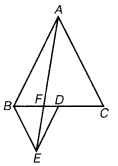Solution.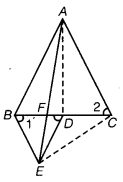Let x be the side of equilateral Δ ABC.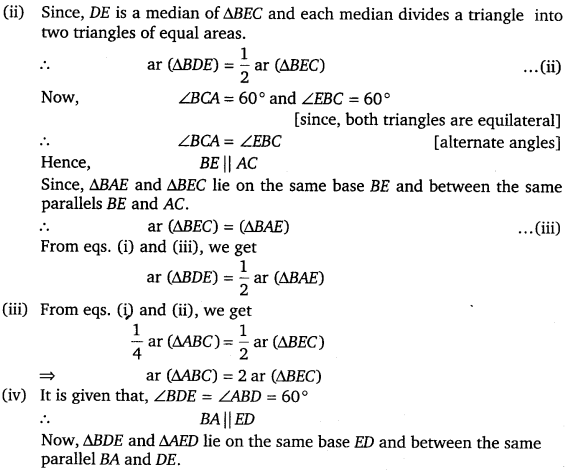Question 6.
Diagonals AC and BD of a quadrilateral ABCD intersect each other at P.
Show that  ar (ΔAPB) x ar (Δ CPD)
= ar (Δ APD) x ar (Δ BPC). [Hint: From A and C, draw perpendiculars to BD].

Solution.Question 7.
P and Q are respectively the mid-points of sides AB and BC of a Δ ABC and R is the mid-point of AP, show that
(i) ar (Δ PRQ) =$\cfrac { 1 }{ 4 }$ ar (Δ ARC)
(ii) ar (Δ RQC =$\cfrac { 3 }{ 8 }$ar (Δ ABC)
(iii) ar (Δ PBQ) = ar (Δ ARC).

Solution.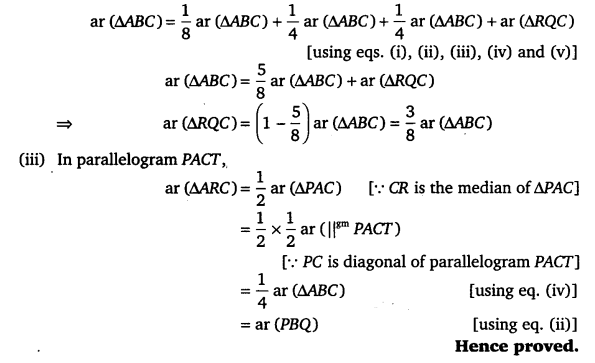Question 8.
In the figure, ABC is a right angled triangle, right angled at A. BCED, ACFG and ABMN are squares on the sides BC, CA and AB, respectively, Line segment AX ⊥DE meets BC at Y. Show that
(i) Δ MBC ≅ Δ ABD
(ii) ar (BYXD) = 2 ar (Δ MBC)
(iii) ar (BYXD) = ar (Δ BMN)
(iv) ΔFCB ≅  AACE
(v) ar (CYXE) = 2 ar (Δ FCB)
(vi) ar (CYXE) = ar (ACFG)
(vii) ar (BCED) = ar (ABMN) + ar (ACFG).

[Note: Result (vii) is the famous Theorem of Pythagoras. You shall term a similar proof by this Theuonern is class X.]Solution.
Given: ABC is a right  angled triangle in which ∠A = 90°. BCED, ACFG and ABMN are squares. Line segment AX ⊥ DE meets BC at Y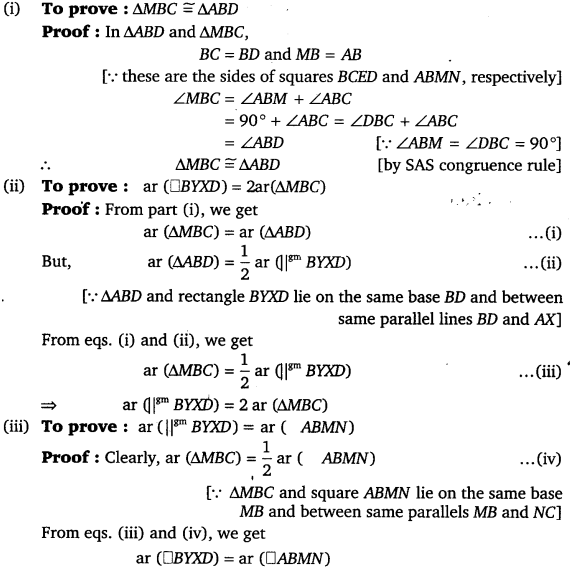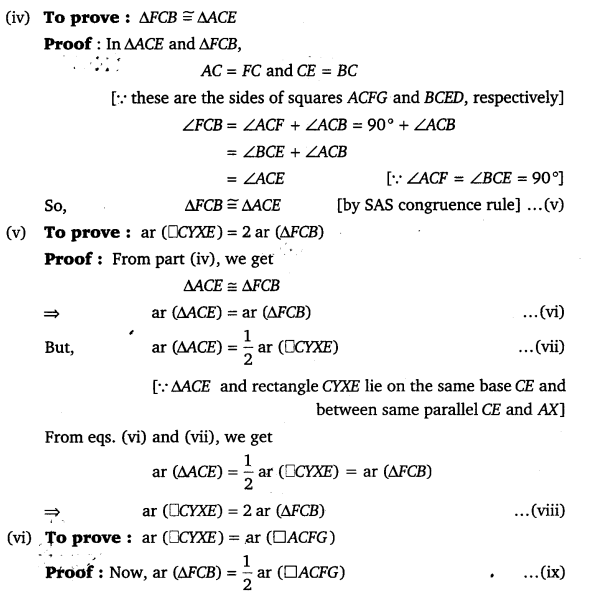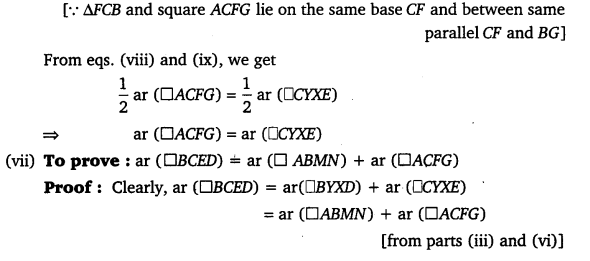#### CLASS 9 MATH NCERT SOLUTION FOR CHAPTER – 10 Circles Ex – 10.1

Lorem ipsum dolor sit amet, consectetur adipiscing elit. Phasellus cursus rutrum est nec suscipit. Ut et ultrices nisi. Vivamus id nisl ligula. Nulla sed iaculis ipsum.

Company Name